JEE  >  BITSAT Physics Test - 6

# BITSAT Physics Test - 6

Test Description

## 40 Questions MCQ Test BITSAT Mock Tests Series & Past Year Papers | BITSAT Physics Test - 6

BITSAT Physics Test - 6 for JEE 2023 is part of BITSAT Mock Tests Series & Past Year Papers preparation. The BITSAT Physics Test - 6 questions and answers have been prepared according to the JEE exam syllabus.The BITSAT Physics Test - 6 MCQs are made for JEE 2023 Exam. Find important definitions, questions, notes, meanings, examples, exercises, MCQs and online tests for BITSAT Physics Test - 6 below.
Solutions of BITSAT Physics Test - 6 questions in English are available as part of our BITSAT Mock Tests Series & Past Year Papers for JEE & BITSAT Physics Test - 6 solutions in Hindi for BITSAT Mock Tests Series & Past Year Papers course. Download more important topics, notes, lectures and mock test series for JEE Exam by signing up for free. Attempt BITSAT Physics Test - 6 | 40 questions in 50 minutes | Mock test for JEE preparation | Free important questions MCQ to study BITSAT Mock Tests Series & Past Year Papers for JEE Exam | Download free PDF with solutions
 1 Crore+ students have signed up on EduRev. Have you?
BITSAT Physics Test - 6 - Question 1

### If the angular speed of rotation of an armature of alternating current generator is doubled, the induced e.m.f. will be

BITSAT Physics Test - 6 - Question 2

### If 20 gm of a radioactive substance due to radioactive decay reduces to 10 gm in 4 minutes, then in what time 80 gm of the same substance will reduce to 10 gm

BITSAT Physics Test - 6 - Question 3

### A radioactive nucleus undergoes α-emission to form a stable element. What will be the recoil velocity of the daughter nucleus if V is the velocity of α-emission and A is the atomic mass of radioactive nucleus

BITSAT Physics Test - 6 - Question 4

Three copper wires have lengths and cross-sectional areas of (l and A), (2l and A/2) and (l/2 and 2A). Resistance will be minimum in

BITSAT Physics Test - 6 - Question 5

When a resistance of 2 Ω is connected across the terminals of a cell, the current is 0.5 amperes. When the resistance is increased to 5 ohm, the current is 0.25 amperes. The internal resistance of the cell is

BITSAT Physics Test - 6 - Question 6

An electron of charge 'E' is liberated from a hot filament and attracted by an anode of potential 'V' volts positive with respect to the filament. The speed of the electron of mass m when it strikes the anode is

BITSAT Physics Test - 6 - Question 7

The de Broglie wavelength λ associated with an electron having kinetic energy E is given by the expression

BITSAT Physics Test - 6 - Question 8

In a car spark coil, an e.m.f. of 40000 V is induced in a secondary when the primary current changes from 4 A to zero in 10μs. Find the mutual inductance between the primary and secondary windings of the spark coil

BITSAT Physics Test - 6 - Question 9

A parallel plate capacitor is first charged and then a dielectric slab is introduced between the plates. The quantity that remains unchanged is

BITSAT Physics Test - 6 - Question 10

An electric dipole placed in a non-uniform electric field experiences

BITSAT Physics Test - 6 - Question 11

A 60 kg weight is dragged on a horizontal surface by a rope to 2 metres. If coefficient of friction is μ=0.5, the angle of rope with the surface is 60o and g=9.8 m/sec2, then work done is

BITSAT Physics Test - 6 - Question 12

If distance between earth and sun become four times, then time period becomes

Detailed Solution for BITSAT Physics Test - 6 - Question 12

According to Kepler's third law (law of periods), the square of the time period of revolution of a planet around the sun is directly proportional to the cube of semi-major axis of its elliptical orbit,
ie, T2 ∝ R3
where T is time taken by the planet to go one around the sun and R is semi-major axis (distance) of the elliptical orbit.
∴ T2 = kR3 .....(i)
where k is constant of proportionality.
When, R becomes 4 times let time period be T'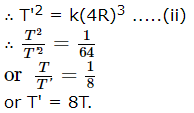So, time period becomes 8 times of previous value.

BITSAT Physics Test - 6 - Question 13

In which of the following process, convection does not take place primarily?

BITSAT Physics Test - 6 - Question 14

The equation of state for 5 g of oxygen at a pressure P and temperature T, when occupying a volume V, will be (where R is the constant)

Detailed Solution for BITSAT Physics Test - 6 - Question 14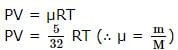BITSAT Physics Test - 6 - Question 15

A potential difference of 2V exists across a potentiometer wire of 2m length. When the potential difference across a 2Ω resistance of a second circuit is measured by this potentiometer wire, it amounts to 5mm balancing length. The current in the second circuit is

Detailed Solution for BITSAT Physics Test - 6 - Question 15

2.5 mA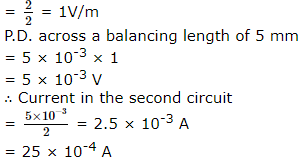BITSAT Physics Test - 6 - Question 16

The effective length of a magnet is 31.4 cm, and its pole strength is 0.5 A-m. If it is bent in the form of a semicircle,then its new magnetic moment will be

BITSAT Physics Test - 6 - Question 17

When the free surface area of a liquid is increased, the surface tension of the liquid

BITSAT Physics Test - 6 - Question 18

If an air-bubble of radius 0.4 mm at a depth h in water having surface tension of 72*10⁻3 N-m⁻1 and density 1000 kg-m⁻3 is in equilibrium then, the value of h is (Take g=10 m-s⁻2)

BITSAT Physics Test - 6 - Question 19

For a constant hydraulic stress on an object, the fractional change in the object's volume (Δ V/V) and its bulk modulus (K) are related as

BITSAT Physics Test - 6 - Question 20

A car is driven for 0.8 s. If the car travelling initially with 36 km − h⁻1 is stopped by the driver after observing a signal by deceleration of 5 m − s−2, then total distance travelled by the car before coming to rest, is

BITSAT Physics Test - 6 - Question 21

A cricketer hits a ball with a velocity 25 m/s at 60o above the horizontal. How far above the ground it passes over a fielder 50 m from the bat (assume the ball is struck very close to the ground)

Detailed Solution for BITSAT Physics Test - 6 - Question 21
The horizontal velocity of the ball will be v cos Θ, or 25 cos 60. This amounts to 12.5 m/s along the x-axis. Then:

50/12.5=4 seconds before the ball passes over the cricketer

The vertical height would be:

h(4)=g/2(4)^2 + 25 sin 60 (4)

=-4.9(16)+ 21.65(4)

=8.2025m as the height of the ball after 4 seconds
BITSAT Physics Test - 6 - Question 22

The maximum speed of a car on a road-turn of radius 30 m, if the coefficient of friction between the tyres and the road is 0.4, will be

BITSAT Physics Test - 6 - Question 23

A person of 70kg mass is lifted by a helicopter with the help of rope hanging from it. If the helicopter is rising with an acceleration of 4 ms-2, the tension in the rope is (g=10 ms-2)

BITSAT Physics Test - 6 - Question 24

The ability of eye to focus near as well as distant objects is called

BITSAT Physics Test - 6 - Question 25

When the potential energy of a particle executing simple harmonic motion is one-fourth of the maximum value during the oscillation, its displacement from the equilibrium position in terms of its amplitude a is

BITSAT Physics Test - 6 - Question 26

Which of the following function represents a simple harmonic motion?

Detailed Solution for BITSAT Physics Test - 6 - Question 26
SHM
The given function is:
sin ωt – cos ωt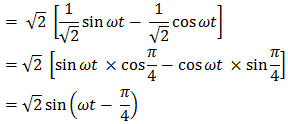This function represents SHM as it can be written in the form: a sin (ωt + Φ)

Its period is: 2π/ω
BITSAT Physics Test - 6 - Question 27

A ray of light is incident at 60o on a prism of refracting angle 30o . The emergent ray is at an angle 30o with the incident ray. The value of refractive index of the prism is

Detailed Solution for BITSAT Physics Test - 6 - Question 27

Angle of prism = 30o = A
Angle of incidence = i1 = 60o
Angle of emergence = i2 = ?
Angle of devation = D = 30o
∴ i2 = A + D - i2 = 30 + 30 - 60 = 0o
⇒ r2 = 0o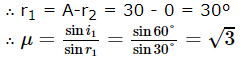BITSAT Physics Test - 6 - Question 28

A person standing on a rotating platform has his hands lowered. He suddenly outstreches his arms. His angular momentum

BITSAT Physics Test - 6 - Question 29

A wheel is rotating at 900 r.p.m. about its axis When power is cut off it comes to rest in 1 minute The angular retardation in rad/s2 is

BITSAT Physics Test - 6 - Question 30

Two rings of the same radius and mass are placed such that their centres are at a common point and their planes are perpendicular to each other. The moment of inertia of the system about an axis passing through the centre and perpendicular to the plane of one of the ring is (mass = and radius = r)

BITSAT Physics Test - 6 - Question 31

The relation between Faraday constant (F), chemical equivalent (E) and electrochemical equivalent (Z) is

BITSAT Physics Test - 6 - Question 32

The densities of two substances are in the ratio 5:6 and their specific heats are in the ratio 3:5 respectively. Then their thermal capacities per unit volume will be in the ratio

BITSAT Physics Test - 6 - Question 33

At N.T.P. one mole of diatomic gas is compressed adiabatically to half of its volume (γ = 1.41). The work done on gas will be

BITSAT Physics Test - 6 - Question 34

Young's modulus of a material has the same units as

BITSAT Physics Test - 6 - Question 35

For diffraction of light, the condition for nth secondary minimum is that path difference is equal to [Here a is the width of slit, n is the order of diffraction, λ is the wavelength of light and θ n gives the direction of nth secondary minimum ]

BITSAT Physics Test - 6 - Question 36

The displacement of the interfering light waves are y₁ = 4 sin ωt and y₂ = 3 sin (ωt + π/2). What is the amplitude of the resultant wave

BITSAT Physics Test - 6 - Question 37

Two waves of intensities I and 4 I superimpose, then maximum and minimum intensities are

Detailed Solution for BITSAT Physics Test - 6 - Question 37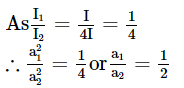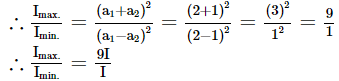BITSAT Physics Test - 6 - Question 38

For production of beats, the two sources must have

BITSAT Physics Test - 6 - Question 39

A quarter horse power motor runs at a speed of 600 r.p.m. Assuming 40% efficiency the work done by the motor in one rotation will be

BITSAT Physics Test - 6 - Question 40

The average resisting force that must act on a 5 Kg mass to reduce its speed from 65cms-1 to 15cms-1 in 0.2s is

## BITSAT Mock Tests Series & Past Year Papers

2 videos|15 docs|70 tests
 Use Code STAYHOME200 and get INR 200 additional OFF Use Coupon Code
Information about BITSAT Physics Test - 6 Page
In this test you can find the Exam questions for BITSAT Physics Test - 6 solved & explained in the simplest way possible. Besides giving Questions and answers for BITSAT Physics Test - 6, EduRev gives you an ample number of Online tests for practice

## BITSAT Mock Tests Series & Past Year Papers

2 videos|15 docs|70 tests